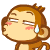Contest - What's the largest number you can think of? | INFJ Forum

# Contest - What's the largest number you can think of?

#### corvidae

##### ohai internets
Donor
I read an interesting article about large numbers, and the ability of computers to calculate them. But before you read the article, I'd like to see you try to tell the biggest number you can think of, within certain rules:

- No use of infinity, infinity + 1, infinity^infinity, etc.
- Must be mathematically definable. So you can't say "the number of water molecules in the Pacific" or "The largest number anyone has ever thought of, plus one"
- Must be describable in 500 characters or less. Use a character count such as http://www.webworldindex.com/countcharacters.htm .
- Don't use Google, Wikipedia, etc. Honor code!

You can make up any funky mathematical notation you want, as long as you can define it within those 500 characters.

Article:

The largest number I can think of (other than infinity) is actually googol. Defined as 10^100.

Googol plex

100^100^100^100^100^100^100^100^100^100^100^100^100^100^100^100^100^100^100^100^100^100^100^100^100^100^100^100^100^100^100^100^100^100^100^100^100^100^100^100^100^100^100^100^100^100^100^100^100^100^100^100^100^100^100^100^100^100^100^100^100^100^100^100^100^100^100^100^100^100^100^100^100^100^100^100^100^100^100^100^100^100^100^100^100^100^100^100^100^100^100^100^100^100^100^100^100^100^100^100^100^100^100^100^100^100^100^100^100^100^100^100^100^100^100^100^100^100^100^100^100^100^100^100^1000

500 characters exactly

13 trillion.Do you mean name and fathom, or actually visualize in my mind? I actually can't visualize anything higher than 10.

The largest number I can think of would be a Googolplex of Googolplexes, multiplied by itself.

y=-ln(1-x) when x=(1/3+1/3+1/3)

y is the large number

thus placing you as close to the divide by zero point (not really, I am not sure what else to call it) as humanly possible (as x = .99 repeating) which is a near infinitely large number.

Wikipediaplex

•enfp can be shy
Ackermann(999...999)
[489 nines]

Edit: Oops. The Ackermann function seems to take two parameters. Lets put in a comma after half of the nines then.

For small values of m like 1, 2, or 3, the Ackermann function grows relatively slowly with respect to n (at most exponentially). For m ≥ 4, however, it grows much more quickly; even A(4, 2) is about 2

Last edited:

7

Avogadro's Number. Especially of socks. Never have to wash socks again!

mooseman has the biggest number so far *and* he gave a hint =D

Just an aside, 99% of things exceed 10^52 or go lower then 10^-52 beyond those limits you start to hit a point of "rediculosness" and things are no longer represented by physical values (I am speaking in terms of physics and chemistry). As an example, one of the smallest numbers used is planks constant which is 6.626x10^-34 which is an obseenly small number. I mean, thats fucking tiny. You really don't get much smaller then that.

Numbers will of course go beyond that in the realms of mathimatics, and maybe computers.

1.47 trillion = 1 470 000 000 000, only 13 digits, that is in the short scale used in America. Where they use the long scale 1.43 trillion is 1 470 000 000 000 000 000, 19 digits, which in the short scale is called 1.47 quintillion.

Last edited: